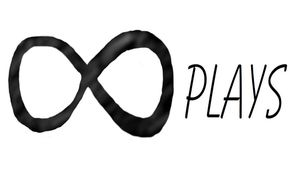By Bob

Using a 3d printer to make useful objects is pretty fun, here is an easy way to make a corrugated riffle with holes for the top section of a high banker.

In Inkscape make a rectangle the height and width you want the riffles to be:

Next go to Extensions>Render>Function Plotter:

Set the values to the values in this picture:

You can adjust the sine wave pattern by changing the End X value.

Then delete the rectangle, go to Path> Stroke to path, select the node tool, highlight the line, convert the segments to lines and save the dxf file:

Next import the file into OpenSCAD, extrude it to the size you want and export the stl file.

``````/*[Holes]*/
hole_number_X=7; //[1:1:100]
hole_number_Y=5; //[1:1:100]
hole_size = 3; //[0:.01:25.4]
hole_shape = 50; //[3:1:50]
hole_spacing_x = 22;//[0:.01:200]
hole_spacing_y = 30;//[0:.01:200]
hole_height=50;//[.1:.01:200]
move_holes_x = .50; //[-20:.01:20]

module holes(){
for(x=[0:hole_number_X-1])
for(y=[0:hole_number_Y-1])
translate([(x-hole_number_X/2+move_holes_x)*hole_spacing_x,(y-hole_number_Y/2+.5)*hole_spacing_y,-5])
linear_extrude(hole_height,convexity=11)
circle(hole_size,\$fn=hole_shape);
}

difference(){
translate([0,0,-1])
import("/home/none3/Desktop/RIFFLES.stl",convexity=11);
holes();
}``````

Just import your stl file where it says import and make adjustments.

I highly recommend learning the function plotter extension in Inkscape, you can make some pretty complex designs in seconds using this method.

## Related ArticlesFollow